Mathematics
Easy

Question

# In the given figure, if the exterior angle is 135o, then ∠P is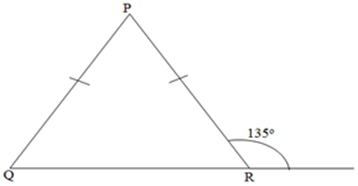## The correct answer is: 90°

Mathematics

### Number of acute angles in a right triangle are ____________.### Number of acute angles in a right triangle are ____________.Mathematics

Mathematics

### These triangles are congruent. If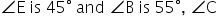is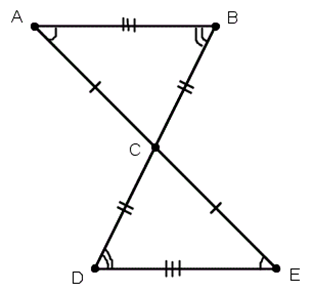Mathematics

Mathematics

Mathematics

###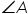is called _________.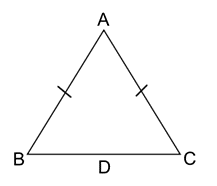Mathematics

Mathematics

Mathematics

### Can the HL congruence theorem be used to prove the triangles congruent? If so, write a congruence statement.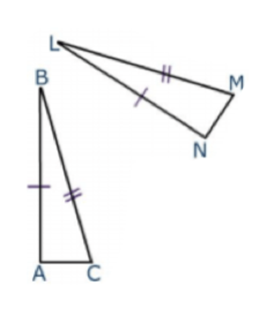Mathematics

Mathematics

Mathematics

### If ∆ ABC and ∆ PQR are to be congruent, then name one additional pair of corresponding parts.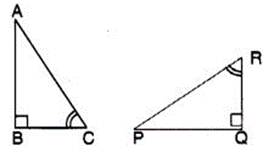Mathematics

### Complete the congruence statement: ∆ BCA =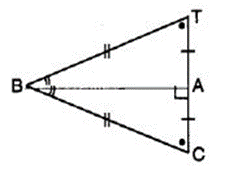Congruent triangles are triangles that have same size and shape. This suggests us that the corresponding sides are equal and corresponding angles are equal.
When two triangles are congruent, all of their corresponding sides and angles are equal.

### Complete the congruence statement: ∆ BCA =Congruent triangles are triangles that have same size and shape. This suggests us that the corresponding sides are equal and corresponding angles are equal.
When two triangles are congruent, all of their corresponding sides and angles are equal.

Mathematics

### We want to show that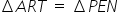. We have to use SAS criterion. We have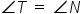, RT = EN, then____________.

SAS congruence is the term which is also known as Side Angle Side congruence. In this, triangles are congruent if any pair of corresponding sides and their included angles are equal in both triangles.

### We want to show that. We have to use SAS criterion. We have, RT = EN, then____________.

SAS congruence is the term which is also known as Side Angle Side congruence. In this, triangles are congruent if any pair of corresponding sides and their included angles are equal in both triangles.

Mathematics

### For two given triangles, ΔABC and ΔPQR, the number of possible matchings are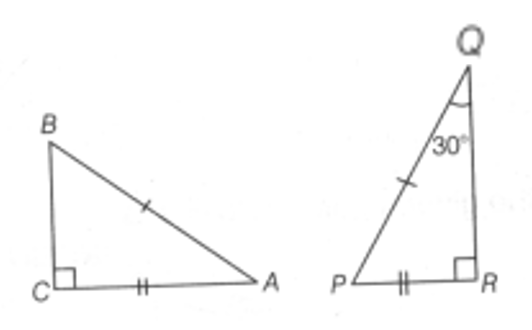#### With Turito Foundation.#### Get an Expert Advice From Turito.